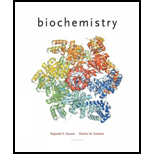# Answers to all problems are at the end of this book. Detailed solutions are available in the Student Solutions Manual, Study Guide, and Problems Book. Calculating the Molecular Weight and Subunit Organization of a Protein From Its Metal Content The element molybdenum {atomic weight 95.95) constitutes 0.0K if the weight of nitrate reductase. If the molecular weight of nitrate reductase is 240,000, what is its likely quaternary structure"?### Biochemistry

6th Edition
Reginald H. Garrett + 1 other
Publisher: Cengage Learning
ISBN: 9781305577206### Biochemistry

6th Edition
Reginald H. Garrett + 1 other
Publisher: Cengage Learning
ISBN: 9781305577206

#### Solutions

Chapter 5, Problem 1P
Textbook Problem

## Answers to all problems are at the end of this book. Detailed solutions are available in the Student Solutions Manual, Study Guide, and Problems Book.Calculating the Molecular Weight and Subunit Organization of a Protein From Its Metal Content The element molybdenum {atomic weight 95.95) constitutes 0.0K if the weight of nitrate reductase. If the molecular weight of nitrate reductase is 240,000, what is its likely quaternary structure"?

Expert Solution
Interpretation Introduction

Interpretation:

The quaternary structure of the nitrate reductase needs to be determined based on its molecular weight and percentage of element molybdenum with atomic weight 95.95 present in it.

Concept introduction:

Nitrate reductase’s quaternary structure can be determined by calculating molecular weight of the molybdenum and its number of moles in nitrate reductase.

From the number of moles of a species and its molar mass, mass can be calculated as follows:

m=n×M

Here, n is number of moles and M is molar mass.

Molecular weight of molybdenum=192 g/mol

Number of moles of molybdenum= 2.00 mol

Nitrate reductase’s quaternary structure consists of two peptide chain and each peptide has one mol of molybdenum. Thus, it is a dimer.

### Explanation of Solution

The Nitrate reductase’s quaternary structure can be determined by calculating number of moles of the molybdenum in a nitrate reductase where one mol of molybdenum is constituted by each peptide.

Molecular weight of molybdenum = nitrate reductase’s molecular weight × molybdenum percentage in nitrate reductase

Therefore,

Molecular weight of molybdenum=240000 g×0.8100=192 g

Molecular weight of molybdenum is used to determine the number of moles of molybdenum in nitrate reductase as follows:

n=mM

Here, m is molecular weight of molybdenum present in nitrate reductase and M is atomic weight or molar mass of the molybdenum.

Putting the values,

n=192 g95.95 g/mol=2 mol

Thus, the number of moles of molybdenum in nitrate reductase is 2.00 mol.

As we know that, one mol of molybdenum is constituted by each peptide. Therefore, nitrate reductase is dimer which is made up of two equivalent peptide chains. And each peptide chain consists of one mole of the molybdenum.

Conclusion

Molecular weight of molybdenum is calculated by using molecular weight of nitrate reductase and molybdenum percentage. The value of molecular weight of molybdenum is needed in finding the number of moles of molybdenum in nitrate reductase. From these calculations, it is determined that the quaternary structure of nitrate reductase constitutes two peptide chains and each chain has one mol of molybdenum.

### Want to see more full solutions like this?

Subscribe now to access step-by-step solutions to millions of textbook problems written by subject matter experts!

### Want to see more full solutions like this?

Subscribe now to access step-by-step solutions to millions of textbook problems written by subject matter experts!

Find more solutions based on key concepts
The formula for ammonium phosphate is _________ .

Introductory Chemistry: A Foundation

Chronic diseases have distinct causes, known as risk factors. T F

Nutrition: Concepts and Controversies - Standalone book (MindTap Course List)

How are length and time used to describe motion?

An Introduction to Physical Science

How can you be sure that giant stars really are Larger than main- sequence stars?

Horizons: Exploring the Universe (MindTap Course List)

Chloroplasts of green algae evolved from _____.

Biology: The Unity and Diversity of Life (MindTap Course List)

Catalytic cracking, fractional distillation, catalytic hydrogenation

Introductory Chemistry: An Active Learning Approach

Why is the following situation impossible? Imagine a copper wire with radius 1.00 mm encircling the Earth at it...

Physics for Scientists and Engineers, Technology Update (No access codes included)